# RD Sharma Solutions for Class 10 Maths Chapter 8 Quadratic Equations Exercise 8.13

Miscellaneous applications of quadratic equations are the key focus in this exercise. The RD Sharma Solutions Class 10 developed by experts at BYJU’S is a prime resource for students to clarify their doubts and concentrate on the weaker areas. Further, students can utilise the RD Sharma Solutions for Class 10 Maths Chapter 8 Quadratic Equations Exercise 8.13 PDF given below for any reference related to the exercise problems.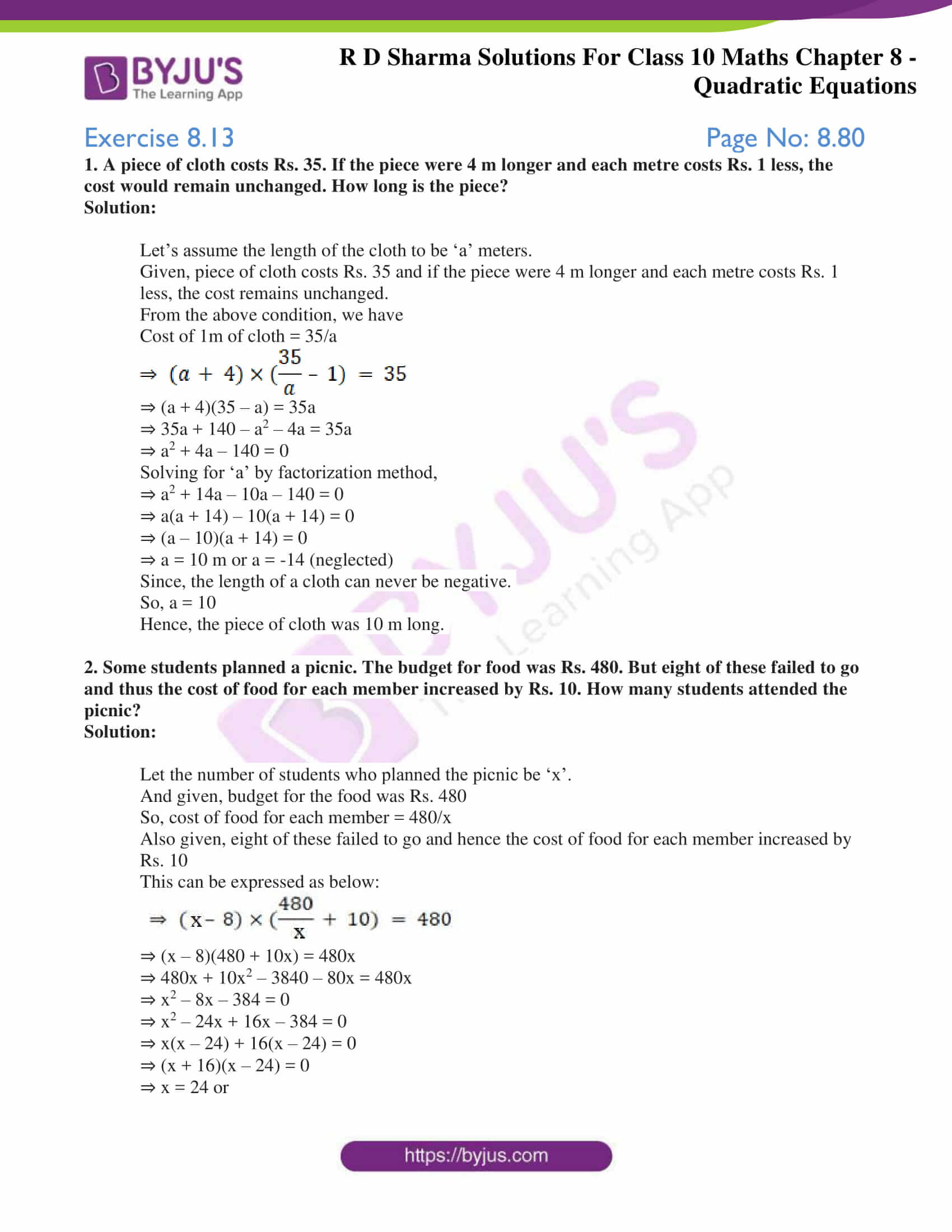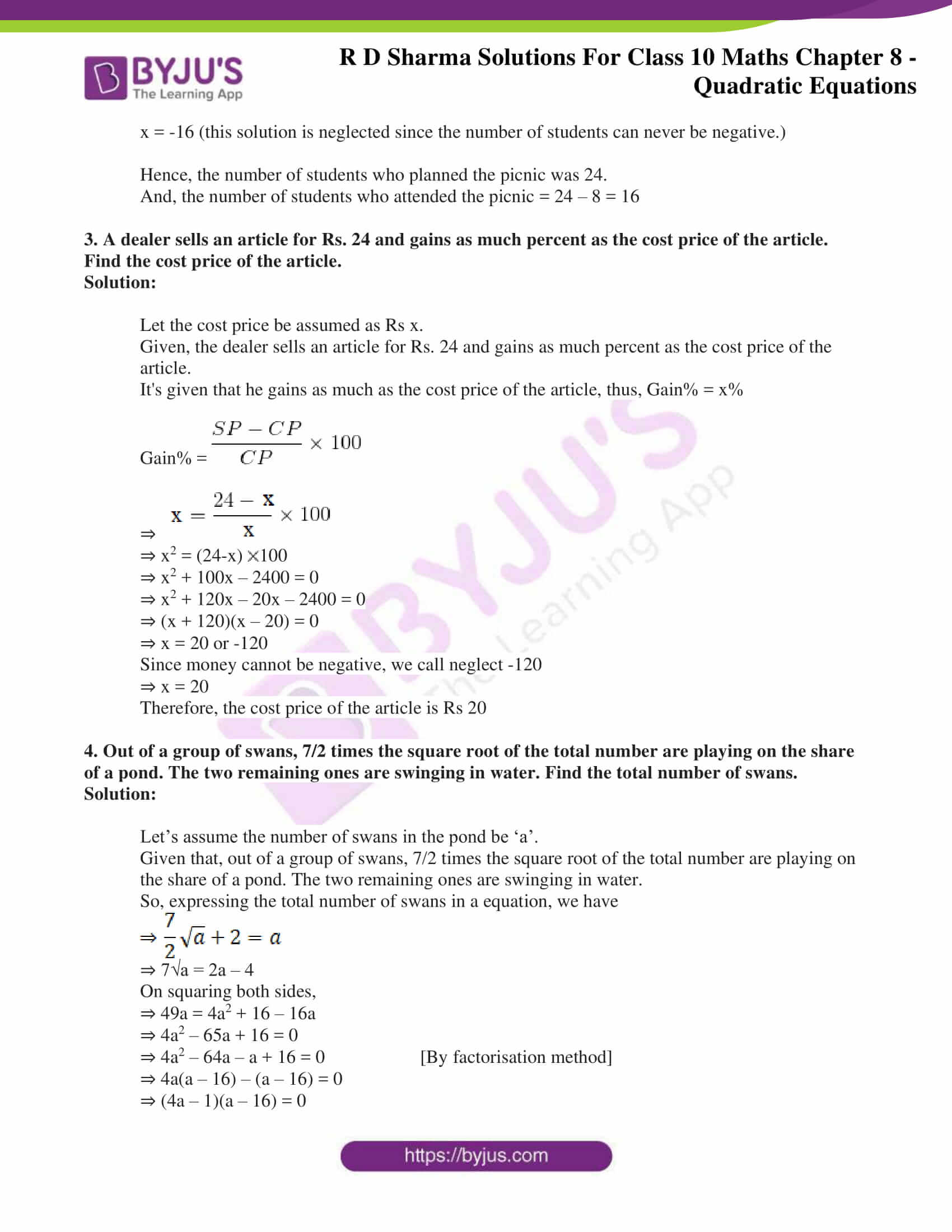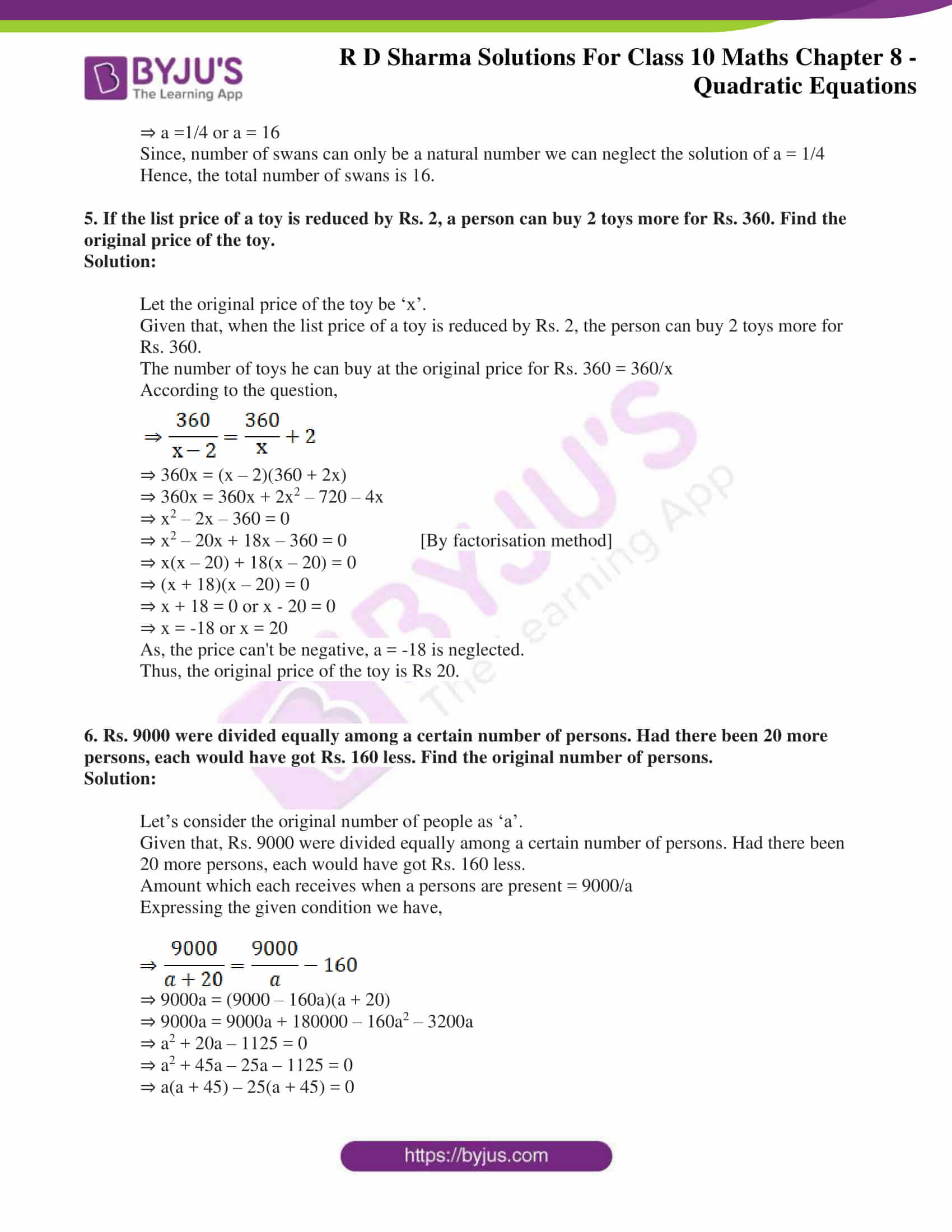### Access RD Sharma Solutions for Class 10 Maths Chapter 8 Quadratic Equations Exercise 8.13

1. A piece of cloth costs Rs. 35. If the piece were 4 m longer and each metre costs Rs. 1 less, the cost would remain unchanged. How long is the piece?

Solution:

Let’s assume the length of the cloth to be ‘a’ meters.

Given, piece of cloth costs Rs. 35 and if the piece were 4 m longer and each metre costs Rs. 1 less, the cost remains unchanged.

From the above condition, we have

Cost of 1m of cloth = 35/a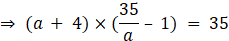⇒ (a + 4)(35 – a) = 35a

⇒ 35a + 140 – a2 – 4a = 35a

⇒ a2 + 4a – 140 = 0

Solving for ‘a’ by factorization method,

⇒ a2 + 14a – 10a – 140 = 0

⇒ a(a + 14) – 10(a + 14) = 0

⇒ (a – 10)(a + 14) = 0

⇒ a = 10 m or a = -14 (neglected)

Since, the length of a cloth can never be negative.

So, a = 10

Hence, the piece of cloth was 10 m long.

2. Some students planned a picnic. The budget for food was Rs. 480. But eight of these failed to go and thus the cost of food for each member increased by Rs. 10. How many students attended the picnic?

Solution:

Let the number of students who planned the picnic be ‘x’.

And given, budget for the food was Rs. 480

So, cost of food for each member = 480/x

Also given, eight of these failed to go and hence the cost of food for each member increased by Rs. 10

This can be expressed as below: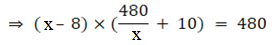⇒ (x – 8)(480 + 10x) = 480x

⇒ 480x + 10x2 – 3840 – 80x = 480x

⇒ x2 – 8x – 384 = 0

⇒ x2 – 24x + 16x – 384 = 0

⇒ x(x – 24) + 16(x – 24) = 0

⇒ (x + 16)(x – 24) = 0

⇒ x = 24 or

x = -16 (this solution is neglected since the number of students can never be negative.)

Hence, the number of students who planned the picnic was 24.

And, the number of students who attended the picnic = 24 – 8 = 16

3. A dealer sells an article for Rs. 24 and gains as much percent as the cost price of the article. Find the cost price of the article.

Solution:

Let the cost price be assumed as Rs x.

Given, the dealer sells an article for Rs. 24 and gains as much percent as the cost price of the article.

It’s given that he gains as much as the cost price of the article, thus, Gain% = x%

Gain% =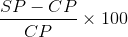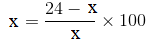⇒ x2 = (24-x)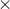100

⇒ x2 + 100x – 2400 = 0

⇒ x2 + 120x – 20x – 2400 = 0

⇒ (x + 120)(x – 20) = 0

⇒ x = 20 or -120

Since money cannot be negative, we call neglect -120

⇒ x = 20

Therefore, the cost price of the article is Rs 20

4. Out of a group of swans, 7/2 times the square root of the total number are playing on the share of a pond. The two remaining ones are swinging in water. Find the total number of swans.

Solution:

Let’s assume the number of swans in the pond be ‘a’.

Given that, out of a group of swans, 7/2 times the square root of the total number are playing on the share of a pond. The two remaining ones are swinging in water.

So, expressing the total number of swans in a equation, we have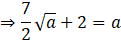⇒ 7√a = 2a – 4

On squaring both sides,

⇒ 49a = 4a2 + 16 – 16a

⇒ 4a2 – 65a + 16 = 0

⇒ 4a2 – 64a – a + 16 = 0 [By factorisation method]

⇒ 4a(a – 16) – (a – 16) = 0

⇒ (4a – 1)(a – 16) = 0

⇒ a =1/4 or a = 16

Since, number of swans can only be a natural number we can neglect the solution of a = 1/4

Hence, the total number of swans is 16.

5. If the list price of a toy is reduced by Rs. 2, a person can buy 2 toys more for Rs. 360. Find the original price of the toy.

Solution:

Let the original price of the toy be ‘x’.

Given that, when the list price of a toy is reduced by Rs. 2, the person can buy 2 toys more for Rs. 360.

The number of toys he can buy at the original price for Rs. 360 = 360/x

According to the question,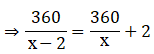⇒ 360x = (x – 2)(360 + 2x)

⇒ 360x = 360x + 2x2 – 720 – 4x

⇒ x2 – 2x – 360 = 0

⇒ x2 – 20x + 18x – 360 = 0 [By factorisation method]

⇒ x(x – 20) + 18(x – 20) = 0

⇒ (x + 18)(x – 20) = 0

⇒ x + 18 = 0 or x – 20 = 0

⇒ x = -18 or x = 20

As, the price can’t be negative, a = -18 is neglected.

Thus, the original price of the toy is Rs 20.

6. Rs. 9000 were divided equally among a certain number of persons. Had there been 20 more persons, each would have got Rs. 160 less. Find the original number of persons.

Solution:

Let’s consider the original number of people as ‘a’.

Given that, Rs. 9000 were divided equally among a certain number of persons. Had there been 20 more persons, each would have got Rs. 160 less.

Amount which each receives when a persons are present = 9000/a

Expressing the given condition we have,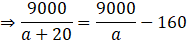⇒ 9000a = (9000 – 160a)(a + 20)

⇒ 9000a = 9000a + 180000 – 160a2 – 3200a

⇒ a2 + 20a – 1125 = 0

⇒ a2 + 45a – 25a – 1125 = 0

⇒ a(a + 45) – 25(a + 45) = 0

⇒ (a – 25)(a + 45) = 0

⇒ a = 25 or a = -45 (neglected as the number of people can never be negative)

Therefore, the original number of people = 25.